### Home > PC > Chapter 12 > Lesson 12.2.3 > Problem12-66

12-66.
1.We want to find a linear transformation that is equivalent to reflecting across the line y = 2x. Homework Help ✎

1. Sketch the graph of y = 2x and find the image of the point (5, 0) when reflected across the line y = 2x.

2. Find a convenient point on the y-axis so that its image when reflected across the line will give integer values for the coordinate of the image. Find the image.

3. Use the points and images you found in parts (a) and (b) to find the linear transformation for reflecting across the line y = 2x.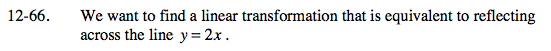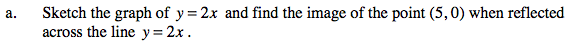Move the red point so that it is reflected.
Note, the segment needs to be perpendicular to the line.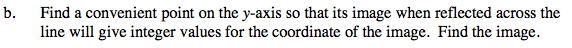Move the red point at (0, 5) so that it is a reflection.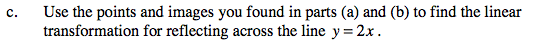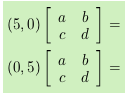Use the eTool below to view the reflection across the line.
Click the link to the right for full version. 12-66 HW eTool